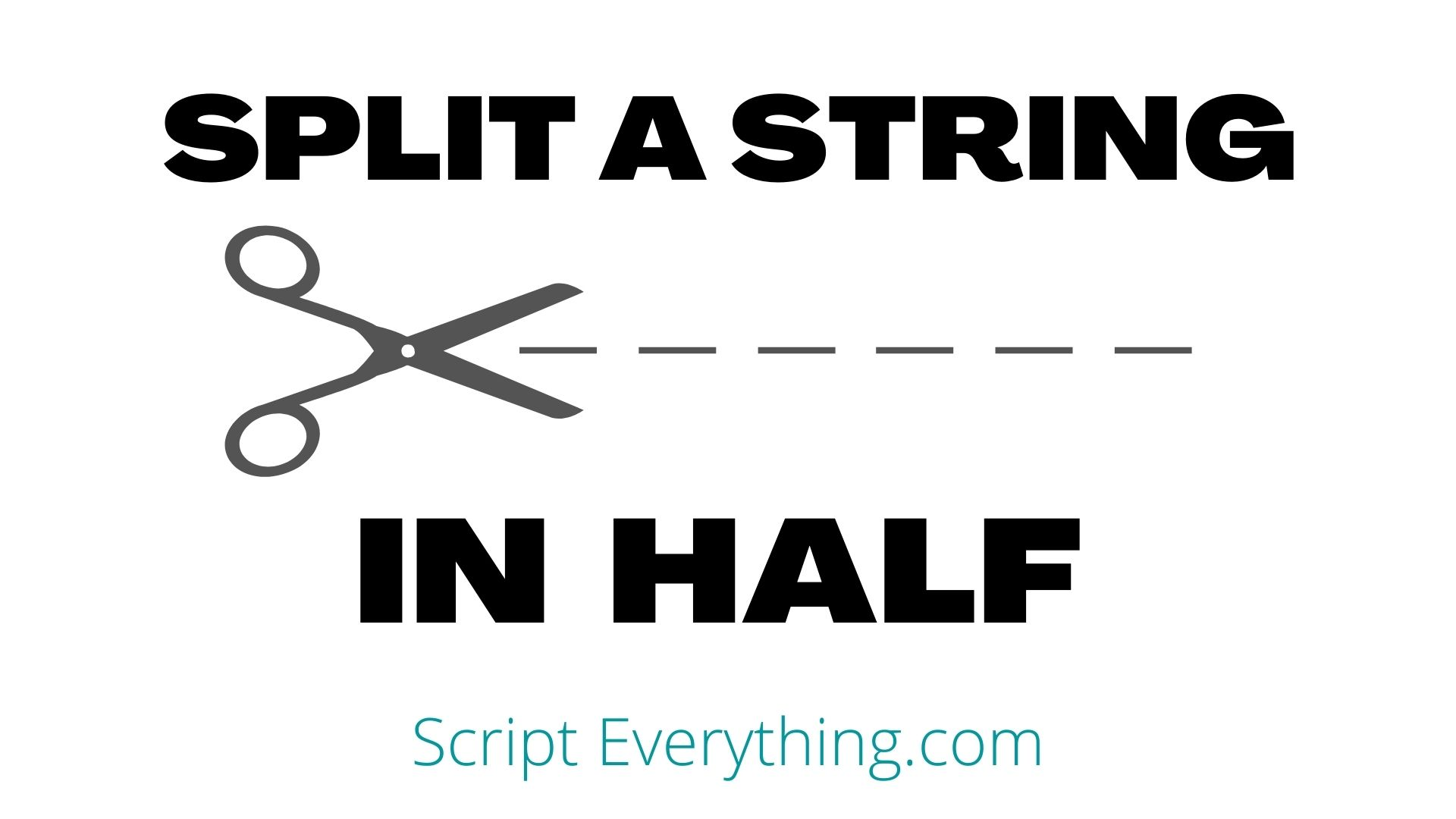# How To Split A String In Half In Python: 1 Line Of CodeHow do you split a string into two equal halves using Python? To tackle this exercise you need to know how you’re going to operate on odd numbered string lengths.

For example, how would you like your code to operate on the string halve . As it has 5 characters you’re going to have 5 possible solutions:

• hal and ve OR ha and lve
• ha, l and ve
• halve OR None

The first solution just placed the middle character to either side giving the extra character to the first half or second half.

The second solution breaks up the string into equal halves, but as there is a middle character it separates this out.

The third solution returns a solution back to the user to imply that the string cannot be halved.are somewhat the same, but give answers to show that the string cannot be halved equally.

Let’s explore one of these solutions and how it can be coded using Python.

## Halve But Put Middle Character To One Side

To halve a string using Python the easiest way is to use the slice operator. The slice operator takes three parameters, with all three being optional.

The slice operator on a string looks something like this:

``my_string[start:stop:step]``

The first parameter ``` start ``` represents the starting index number to begin the capture from the original string ``` my_string ``` and is inclusive of that capture. The second parameter ``` stop ``` represents the ending index number to stop capture from the original string and is exclusive of that capture. Finally, the third parameter ``` step ``` is the jump capture from ``` start ``` to ``` stop ``` .

If the ``` start ``` value is empty it is assumed the capture begins at the first character in the string. If the ``` stop ``` value is empty it is assumed the capture stops at the last character in the string. Finally, if the ``` step ``` value is empty it is assumed the jump capture is 1.

The next step with this halving problem is to work out how to handle odd-numbered characters from the string.

If a string has an even number of characters then the halving is quite easy, however, if the number of characters in the string is odd then you will need to round either up or down.

### Is Number Of Characters Odd Or Even?

If you have a string that contains an odd amount of characters you would need to round down that number as you cannot capture half of a character.

Thankfully, by using the double slash operator in Python you can round down a positive number.

``````>>> my_string = "Oddly"
>>> len(my_string)
5
>>> len(my_string)/2
2.5
>>> len(my_string)/2 // 1
2.0
>>> int(len(my_string)/2 // 1)
2``````

As you can see from the above operations by using the double slash operator on a positive number the number has a floor division applied when that floor division is done against 1.

To remove the decimal place I wrapped everything in the built-in ``` int(n) ``` function.

To obtain the two halves of our string the operation can now be wrapped into something like this:

``````>>> my_string = "Oddly"
>>> half = int(len(my_string)/2 // 1)
>>> my_string[:half], my_string[half:]
('Od', 'dly')
>>> my_string = "Evenly"
>>> half = int(len(my_string)/2 // 1)
>>> my_string[:half], my_string[half:]
('Eve', 'nly')``````

As you can see from the above examples the split in half operation works very well for even-numbered words, but for odd-numbered words it tries to do its best and adds an additional character to the second half.

Therefore, a solution if used as a single one-liner piece of Python code would look something like this:

``````>>> my_string = "Oddly"
>>> my_string[:(x:=int(len(my_string)/2//1))], my_string[x:]
('Od', 'dly')
>>> my_string = "Evenly"
>>> my_string[:(x:=int(len(my_string)/2//1))], my_string[x:]
('Eve', 'nly')``````

The code example above uses the walrus operator to prevent doubling the operation where the calculation of half the string is performed: ``` int(len(my_string)/2//1 ``` . By assigning the value from this to a variable ``` x ``` I can reuse this value later in the one-liner statement.

## Summary

To cut a string into halves use the following operation which returns a tuple of each half: ``` my_string[:(x:=int(len(my_string)/2//1))], my_string[x:] ``` where ``` my_string ``` is the variable containing the string to be halved.

By performing this operation you would have discovered several handy operators in Python: slice operator , floor division operator , and the walrus operator .

Author of scripteverything.com, Ryan has been dabbling in code since the late '90s when he cut his teeth by exploring VBA in Excel when trying to do something more. Having his eyes opened with the potential of automating repetitive tasks, he expanded to Python and then moved over to scripting languages such as HTML, CSS, Javascript and PHP. When he is not behind a screen, Ryan enjoys a good bush walk with the family during the cooler months, and going with them to the beach during the warmer months.